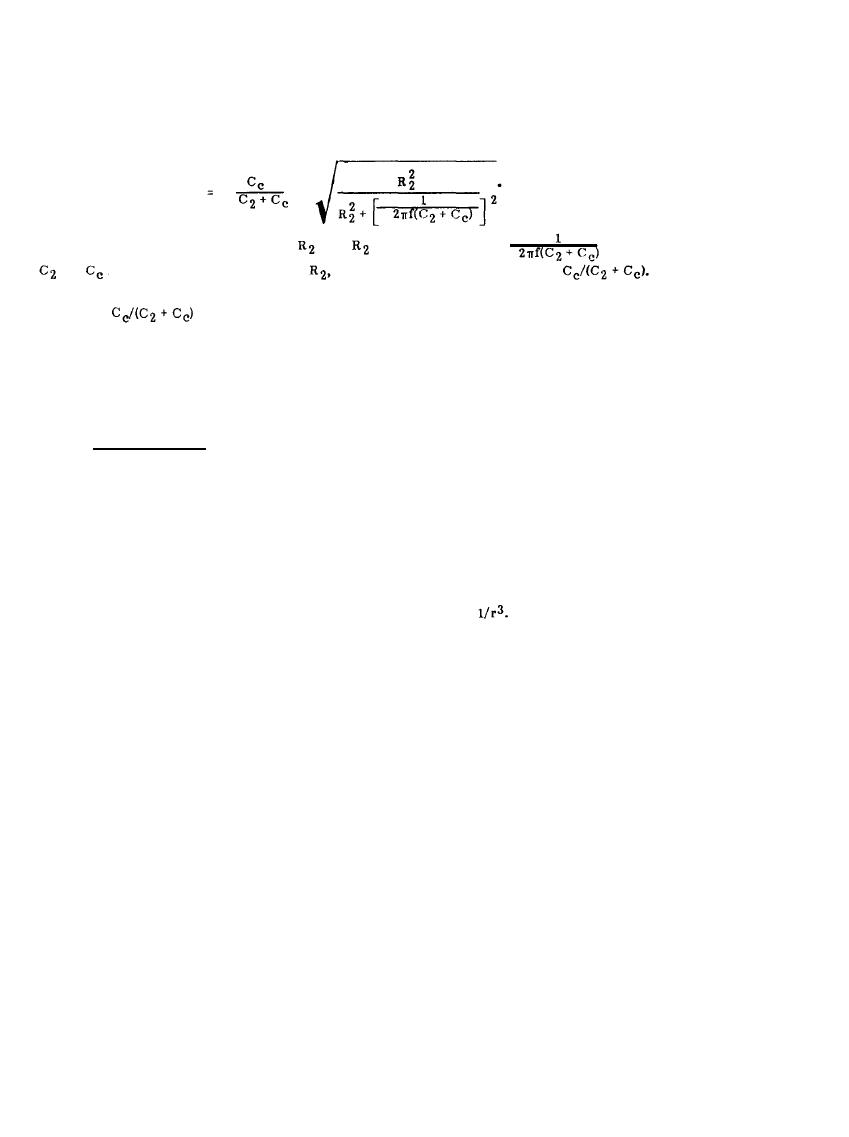Far-Field CouplingCustom SearchMIL-HDBK-419A
(6-20)
This ratio increases almost linearly with
until
approaches the value
; i.e., the reactance of
and
in parallel. For larger values of
the ratio asymptotically approaches
The behavior
of this voltage ratio with frequency is illustrated in Figure 6-11. The ratio is zero at dc and asymptotically
approaches
as the frequency is increased. Equation 6-20 and Figure 6-11 illustrate again that the
voltage capacitively coupled into the susceptible circuit increases with an increase in the total resistance of the
circuit and with an increase in frequency. Resonances can occur and change the amount of capacitive coupling
if the impedance of the susceptible circuit contains inductive reactance, but such resonances usually only
produce noticeable effects at higher frequencies.
6.2.2.4 Far-Field Coupling.
Radiation is the means by which energy escapes from a conductor and propagates into space. The conductor
does not have to be specifically designed to radiate energy; it may be any current carrying conductor; e.g., a
signal line, a power line, or even a ground lead.
Algebraic expressions for the electromagnetic fields surrounding a current carrying conductor are usually
expressed as the sum of three terms. Each term is inversely proportional to a power of the distance, r, from
the conductor, i.e., each term is proportional to either 1/r, 1/r2, or
Close to the conductor (near field),
2
3
t h e 1 / r a n d 1 / r  components dominate and the electromagnetic energy oscillates between the space
surrounding the conductor and the conductor itself; zero average energy is propagated by the near field terms.
Outside the near field region, the l/r term predominates. In this far field region, radiated energy that has
escaped is propagating away from the "antenna" through space. The mechanism of energy radiation can be
visualized (6-3) by considering the finite time required for the electromagnetic fields to propagate between two
points in space. Current flows through an antenna at the frequency of the applied signal, and the polarity of the
field produced by this current is reversed at this same frequency. When a positive charge is present at one end
of the an equal but, negative charge is present at the other end and an electric field in the vicinity of
the antenna will be established between the charges. As the current changes direction, the charges will reverse
positions; the electromagnetic field will collapse and be re-established in the opposite direction. If the
frequency of the applied signal is low, sufficient time will exist between reversals for practically all the energy
stored in the field to be returned to the circuit and very little radiation will occur. If, however, the frequency
is high and the charges reverse quickly, a field in the opposite direction is formed near the wire before a
substantial amount of the field energy can return to the circuit. This part of the field is thus separated from
the antenna and propagates outward through space as an electromagnetic wave.
6-14Integrated Publishing, Inc.